PRIMARY

2 - 6

SECONDARY

7 - 10

SENIOR

11 - 12

Home

# Quotient Rule

Theory & Practice Questions.

## Lesson

#### [Formula]

If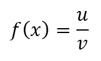where u and v are both functions of x, then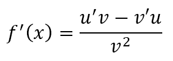## Example

Differentiate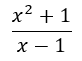## Solution

Recall that ifwhere u and v are both functions of x, thenu = x² + 1      v = x-1

u' = 2x           v' = 1

Using this, we get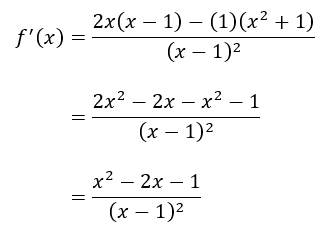## Question

Differentiate the function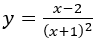## Explanation

Use the quotient rule of differentiation, which says that ifwhere u and v are both functions of x, thenu = x - 2      v = (x+1)²

u' = 1           v' = 2(x+1)

Using the formula, we have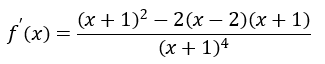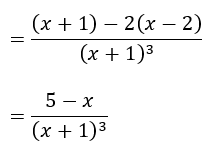School Year